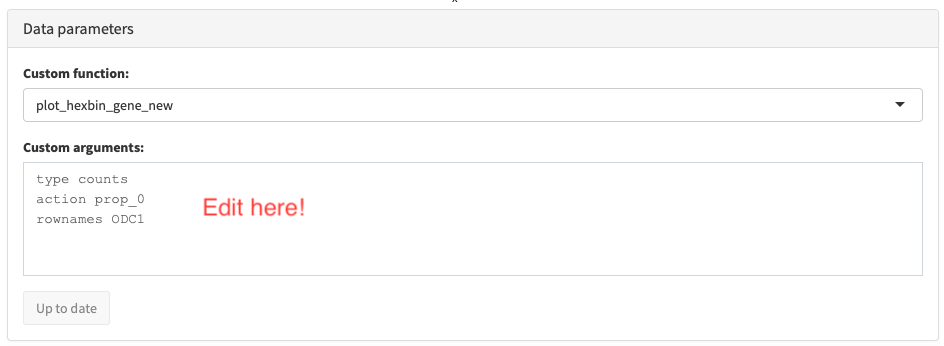# Communicating results with schex and shinydashboard or iSEE

library(shiny)
library(Seurat)
library(ggrepel)
library(shinydashboard)
library(schex)
library(iSEE)

## Setting up for a simple shiny dashboard or iSEE instance

Interrogating your data is often easier when being able to do it interactively on the fly. With the packages shiny and shinydashboard it is simple to create an interactive dashboard for exactly that purpose. Here we will work with the single cell data from peripheral mononuclear cells (PBMCs) stored in the pbmc_small Seurat object (which can be found in the Seurat package.)

First we create a the hexagon cell representation and also make a data frame to store the position of cluster labels.

pbmc_small <- make_hexbin(pbmc_small, nbins = 10, dimension_reduction = "PCA")
df_label <- make_hexbin_label(pbmc_small, "RNA_snn_res.0.8")

## The shiny dashboard

Now it is pretty easy to create a simple shiny dashboard which allows you to visualize the gene expression of every gene in the dataset. After running the function, the application is initialized by running app.

app <- shinyApp(
server= function(input, output){

output$all_genes <- renderUI({ selectInput(inputId = "gene", label = "Gene", choices = rownames(pbmc_small)) }) output$plot1 <- renderPlot({
plot_hexbin_meta(pbmc_small, "RNA_snn_res.0.8", action="majority",
title="Clusters") + guides(fill=FALSE) +
ggrepel::geom_label_repel(data=df_label,
aes(x=x, y=y, label=label), colour="black",
label.size=NA, fill=NA)

})

output$plot2 <- renderPlot({ plot_hexbin_feature(pbmc_small, type=input$type, feature=input$gene, action=input$action, title=input$gene) }) }, ui= dashboardPage(skin = "purple", dashboardHeader(), dashboardSidebar( uiOutput("all_genes"), radioButtons("type", "Type of expression:", c("Raw" = "counts", "Normalized" = "data")), radioButtons("action", "Summarize using:", c("Proportion not 0" = "prop_0", "Mean" = "mean", "Median" = "median")) ), dashboardBody( fluidRow( box(plotOutput("plot1", width = 450, height=400), width=6), box(plotOutput("plot2", width = 500, height=400), width=6)) ) ) ) ### Customizing your own shiny dashboard If you want to add functionality to your shiny dashboard, I would suggest the following resources: ## An iSEE instance The iSEE package provides another way of generating an interactive session in order to interrogate your data in a web browser. However in order to use schex plots you need to provide a custom function that works on an object of the SummerizedExperiment class. The SingleCellExperiment class inherits all features from this class and can thus be used. pbmc_small <- as.SingleCellExperiment(pbmc_small) pbmc_small <- make_hexbin(pbmc_small, nbins=10, dimension_reduction = "PCA") plot_hexbin_gene_new <- function(sce, rows=NULL, rownames=character(0), columns=NULL, type="logcounts", action="prop_0"){ plot_hexbin_feature(sce, type=type, feature=rownames, action=action) } Simply now tell iSEE about schex, using the following. After running the functions, the application is initialized by running app. schex_plot_gene <- customDataPlotDefaults(pbmc_small, 1) schex_plot_gene$Function <- "plot_hexbin_gene_new"
schex_plot_gene$Arguments <- "type counts\naction prop_0\nrownames ODC1" schex_plot_gene$ColumnSource <- "NULL"
schex_plot_gene$RowSource <- "NULL" schex_plot_gene$DataBoxOpen <- TRUE

app <- iSEE(
pbmc_small,
customDataArgs=schex_plot_gene,
initialPanels=DataFrame(
Name=c("Custom data plot 1"),
Width=c(12)),
customDataFun=list(plot_hexbin_gene_new=plot_hexbin_gene_new)
)

Unfortunately in the iSEE application plots cannot be changed to display different genes as conveniently as with shinydashboards. If you want to diplay a different gene you will need to edit the following box:For example by typing

type counts
action prop_0
rownames CD19

you can change the plot to display the expression of CD19.